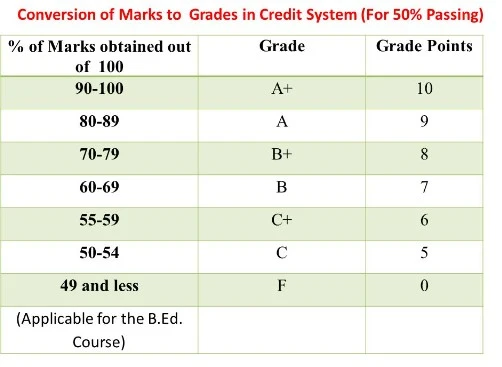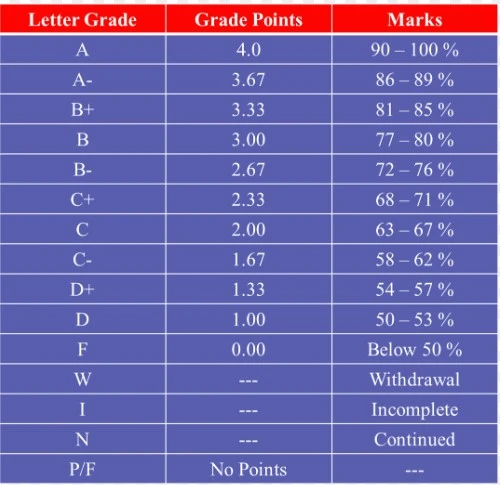CGPA to Marks Calculator

## What Is CGPA (Cumulative Grade Point Average)?

CGPA is an abbreviation for Cumulative Grade Point Average. The term is used to denote the overall average performance of students throughout their academic program in a year. Unlike regular percentages that are often used to denote academic performance, CGPA is denoted by a number based on different grading systems.

While it is general practice to get percentage from GPA and CGPA, there are times when you might want to know the exact marks from your CGPA score. In fact, some institutions look for marks rather than Cumulative Grade Point Average. In such a situation, knowing how to convert CGPA to marks can be useful. CGPA is often used to assess a student's overall academic achievement for a given subject area.

For example, in the Indian Grading System, if you get an ‘A grade’ in a subject, then the marks obtained would range between ‘90-95’ and your CGPA calculated would be ‘9’. For other schools, they may use a grade point system. For example, “Grade Point 9” is assigned to a range of 90-95 points in accordance with the CGPA system. In any case, most academic institutions make their CGPA conversion table available online for students to use.

CGPA is often important for students in the final year of high school as it is used in their matriculation process for higher education. It is especially important for students who are seeking academic opportunities in foreign colleges and universities.

## The CGPA Formula

While it is general practice to get percentage from GPA and CGPA, there are times when you might want to know the exact marks from your CGPA score. In fact, some institutions look for marks rather than Cumulative Grade Point Average. In such a situation, knowing how to convert CGPA to marks can be useful.

As per the CBSE, the formula to execute this conversion is: Percentage of Marks = 9.5 x CGPA.

This means that in order to convert your CGPA to marks, you need to first convert it into a percentage, by multiplying CGPA to 9.5 (explained below).

## How To Calculate Your Total Marks?To calculate your total marks, you need to multiply your percentage divided by 100 to the total marks for all subject. Let’s take an example. If you received a 6 CGPA in 12th, then your total percentage of marks would be, 6×9.5= 57%.

#### Here is how you calculate total marks:

Here is how you calculate total marks:

• Multiply CGPA by 9.5. You get your total percentage
• Now divide total percentage by 100 and multiply it with total marks.
• End result is the marks you obtained

Now, if you have a total of say 6 subjects with 100 as total marks each, then your marks in 12th would be: (57/100) x 500= 285. Therefore, 6 CGPA would be 285 marks.

Here’s the example in a simple format:

• 6*9.5=57
• (57/100)*total marks
• Let total marks be 500
• (57/100)*500=285

## How To Calculate Your CGPA For The Semester?

Your CGPA is just the average of your CGPA scores for all subjects for the semester. To calculate your CGPA for the semester, add all your grade points for all your subjects and then divide it by the total number of subjects. The result will be your CGPA for that particular semester.

## CGPA And The CBSE

The CBSE (Central Board of Secondary Education) examinations is an important centralized public board exam for students in India. The exam is taken by all students attending schools associated with the CBSE. With CBSE exams, students can be graded on their performance in their courses using the grading method, which is believed to be superior to the well-known traditional mark system.

The examination is taken after class 10 and the result is important for admission into college or university. The results of the exam or a student’s marks are crucial when a student is seeking academic opportunities in foreign colleges and universities.

## Why Multiply By 9.5?

To arrive at the 9.5 multiplier, the CBSE referred to the results of the previous 5 years and then found average marks of all the students who had their scores in the top percentile of 91 to 100.

From this percentile range, the best average is 95 marks which equates to an A1 grade or 91-100 marks which is 10 GPA. As such, the CBSE divided 95 by to to get the 9.5 multiplier fro CGPA to marks calculations.

## Advantages of the CGPA System

The major benefit of the CGPA system is that it is cumulative. With CGPA, the real academic score and its corresponding marks are not accounted for on the official transcripts of student.

This means that if a student is weak in a particular area and strong in others, their net performance will determine their CGPA. As such, CGPA denotes a student’s overall performance in the academic year.

Instead of worrying about a specific letter grade, CGPA allows the students to see a holistic view of their performance considering weaknesses and strengths.

Importantly, your CGPA is an important prerequisite for higher education or even getting a job in many cases.

Another major advantage of the CGPA system is that is it more all-encompassing. It measures a student’s knowledge and abilities via school based assessments, major projects and their performance in major exams.

The previous percentage system was less inclusive and simply considered performance in exams. The percentage system is indicative of a student’s ability to study and regurgitate for exams. However, with CGPA, the exam board can determine whether a student is actually able to understand the concepts that they are learning.

#### Easy to use

The calculation i relatively simple

#### Important For Matriculation

The score is important for acceptance to major colleges

#### Cummulative

Gives a better overview of a student;s overall academic performance## FAQs

#### What is maximum marks CGPA?

The CGPA between 9.5 to 10 Grade Point is considered as the maximum marks CGPA. Ths works out to be 90.25 Marks to 95 Marks

#### What is the CGPA of 73%?

To calculate CGPA of 73%, we can simply multiply it by 9.5, which will be 7.68 CGPA.